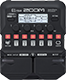# 1231121

Discussion in 'Zoom G1/G1X Four' started by Yan Alves, Aug 3, 2019.

1. 1231121Device: Zoom G1 Four
Firmware: 1.10

Name on device: SLASH
Optimized for: Phones/Speaker

Effects chain:Without ZNR

Effect: "ParaEQ" (Filter), active - "yes"
"Frequency" = 125Hz
"Q" = 1.0
"Gain" = 4.0
"Volume" = 78

Effect: "ParaEQ" (Filter), active - "yes"
"Frequency" = 630Hz
"Q" = 1.0
"Gain" = 1.0
"Volume" = 80

Effect: "MetalWRLD" (Overdrive / Distortion), active - "yes"
"Gain" = 80
"Bass" = 72
"Treble" = 26
"Volume" = 74

Effect: "Gt GEQ" (Filter), active - "yes"
"160" = 3.0
"400" = 2.0
"800" = -2.0
"3.2k" = -1.0
"6.4k" = 3.0
"12k" = 0.0
"VOL" = 68

Effect: "TapeEcho" (Delay), active - "no"
"Time" = 50
"F.B." = 0
"Mix" = 0
"Tail" = Off

Patch Volume: 80